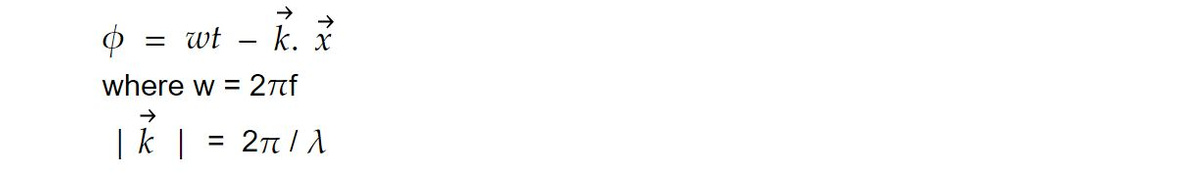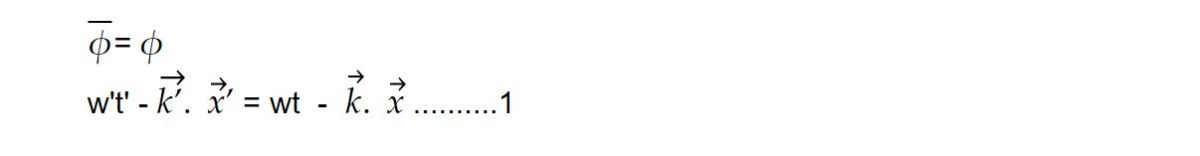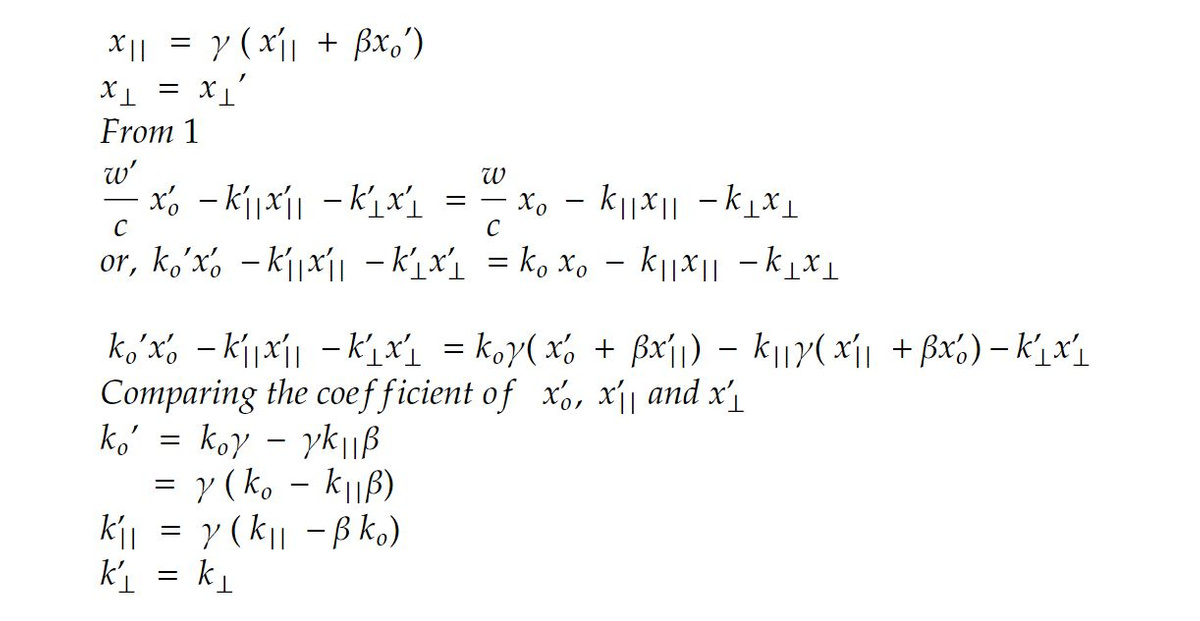# Phase Invariance

Phase represents state of vibration of a curve at given instant. It is expressed in terms of radian. It is given byPhase is an invariant quantity.

Phase lapsed at a point is proportional to number of crest crossing the point. So, knowing phase at a point is a counting process that how many crest have crossed point.

Counting process does not depend on system of reference.From this we can final transformation law for wave vector.

Equation 1 can be written asThe Lorentz transformation for xll and x⟂ areγ is the Lorentz factor, which depends on the relative velocity (β) between the frames. These are Lorentz transformation for the wave vector. Ko, k₁, k₂, and k₃ are called four wave vector. The equation k'⟂ = k⟂ represents the conservation of the component of the wave vector perpendicular to the motion of the frame during the Lorentz transformation. This conservation ensures that the wave vector's component in the direction perpendicular to the relative motion remains unchanged as observers in different inertial frames describe the same physical phenomenon. This concept is important in relativistic physics, particularly when analyzing the behaviour of waves in different reference frames.

This note is a part of the Physics Repository.